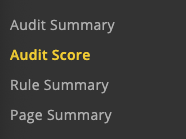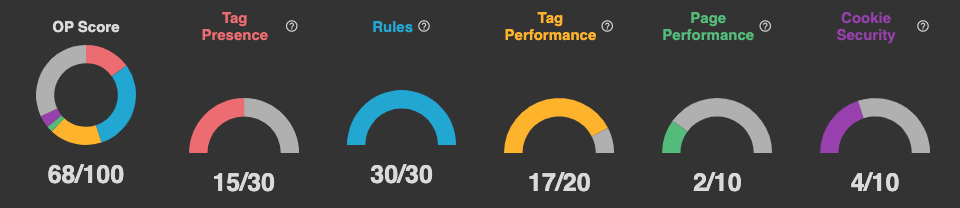# Audit Score

### Overview

The  Audit Score report is designed to give you a pulse on your website's response to best practices (as defined by ObservePoint).Audit Score (100 points)

This is the total score and a sum of the individual score factors which are described in detail below. You can see details regarding each score factor by hovering over the ? icons.

Tag Presence (30 points)

This metric scores you on the implementation rate of your Primary Tags. The score is calculated by taking the average tag presence % of all Primary Tags and multiplying by 30 (the factor weight).

Note: If no Primary Tags are configured, all tags are considered.

Rules (30 points)

This metric scores you on the % of total pages that have failed rules. The score is calculated by taking the total number of pages with rule failures divided by total pages scanned and multiplying by 30 (the factor weight).

Note: If not rules are applied, the score defaults to 30. We strongly recommend applying rules to all audits.

Tag Performance (20 points)

This metric scores you on the load time of your Primary Tags. The score is calculated by taking the % of tags that load under 500ms and multiplying by 20 (the factor weight).

Note: If no Primary Tags are configured, all tags are considered.

Page Performance (10 points)

This metric scores you on the page load time across your site(s). The score is calculated by taking the % of pages that load under 3 seconds and multiplying by 10 (the factor weight).